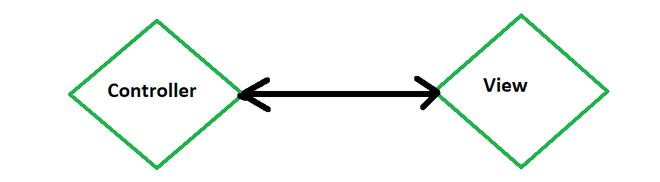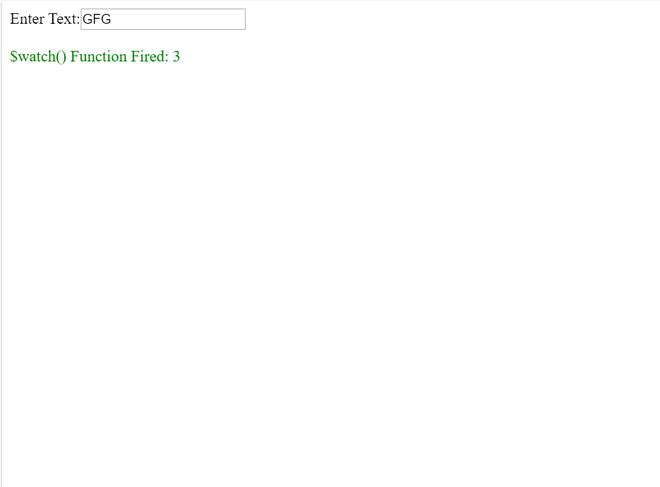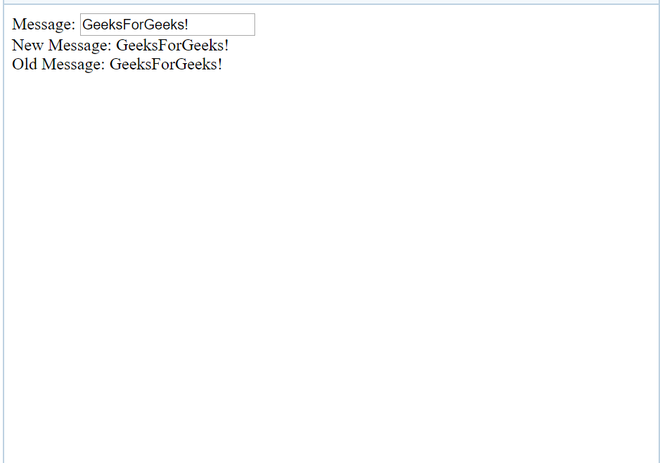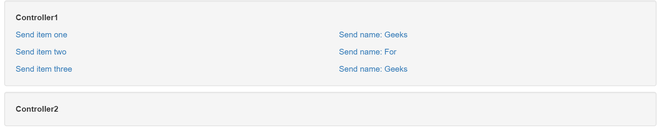# How to watch service variables ?

• Last Updated : 10 Sep, 2020

\$scope acts as a built-in object in AngularJs.

It consists of application data and methods. The \$scope is acts as a link between a controller and view (HTML).\$scope is used to transfer the data.

\$watch:

Scope in AngularJs object has \$watch event which comes into picture when the model property gets changed or altered.

When a data binding is created from some point of program in the view to a variable on the \$scope object, a watch is created internally in AngularJs.

The digest gets called multiple times, when we \$watch() a function.

Every time when we bind the UI, We insert \$watch in \$watch list.

```User: <input type="text" ng-model="user" />
```

Example:

 ` ` `<``html``> ` `    ``<``head``> ` `        ``<``title``> ` `            ``AngularJs \$watch() Function with GFG ` `        `` ` `        ``<``script` `src``= ` `"https://ajax.googleapis.com/ajax/libs/angularjs/1.4.8/angular.min.js"``> ` `      `` ` `        ``<``script` `type``=``"text/javascript"``> ` `            ``var app = angular.module("watchApp", []); ` `            ``app.controller("watchCtrl", function (\$scope) { ` `                ``\$scope.count = -1; ` `                ``\$scope.\$watch( ` `                  ``"txtval", function (newval, oldval) { ` `                    ``\$scope.count = \$scope.count + 1; ` `                ``}); ` `            ``}); ` `        `` ` `    `` ` `    ``<``body``> ` `        ``<``div` `ng-app``=``"watchApp"`  `             ``ng-controller``=``"watchCtrl"``> ` `            ``Enter Text:<``input` `type``=``"text"`  `                              ``ng-model``=``"txtval"` `/> ` `          ``<``br` `/> ` `            ``<``br` `/> ` `            ``<``span` `style``=``"color: Green;"``> ` `              ``\$watch() Function Fired: {{count}} ` `        `` ` `    `` ` ` `\$watch in AngularJs is a service.

It is used to keep the track of the changes on specific properties within a given scope. It is similar to an event listener. e changes are made in the value of specified variables.

When we register a watch we pass two functions as parameters to the \$watch() function.These functions are:

• A value function
• A listener function

Example:

```\$scope.\$watch(function() {}, //value function
function() {}  //listener function
);```

A watcher can change in responses on:

1. Timeouts
2. UI
3. Complex asynchronous computations performed by web workers
4. Ajax calls

Methods Used: \$scope.\$watchGroup

\$watchGroup() is a shortcut to set up watchers with the same callback, passing an array of watchExpressions.

\$scope.\$watchGroup([‘obj.a’, ‘obj.b.bb’, ‘letters’], function(newValues, oldValues, scope) {
//…
});

\$scope.\$watchCollection is a shortcut to watch arrays or objects. In arrays, the listener is called when any of the elements are replaced, deleted, or added.

The \$watch keeps track of the variable. This function takes two arguments:

1. new value

\$scope.\$watch(‘expression’, function (newvalue, oldvalue) { //expression parameter
//Code
});

2. old value

\$scope.\$watch(function () {}, function (newvalue, oldvalue) { //Function Parameter
// Code
});

Example:

 ` ` `<``html``> ` `<``head``> ` `    ``<``script` `src``= ` `"https://ajax.googleapis.com/ajax/libs/angularjs/1.3.16/angular.min.js"``> ` `   `` ` ` ` `<``body` `ng-app``=``"myNgApp"``> ` ` `  `    ``<``div` `ng-controller``=``"myController"``> ` `        ``Message: <``input` `type``=``"text"` `                        ``ng-model``=``"message"` `/>  ` `        ``<``br` `/> ` `        ``New Message: {{newMessage}} <``br` `/> ` `        ``Old Message: {{oldMessage}}  ` `    `` ` `    ``<``script``> ` `        ``var ngApp = angular.module('myNgApp', []); ` `     `  `        ``ngApp.controller('myController', function (\$scope) { ` `            ``\$scope.message = "GeeksForGeeks!"; ` ` `  `            ``\$scope.\$watch( ` `'message', function (newValue, oldValue) { ` `                ``\$scope.newMessage = newValue; ` `                ``\$scope.oldMessage = oldValue; ` `            ``}); ` `        ``}); ` `    `` ` ` ` ``Others:

\$digest()

We call the the \$digest() function when AngularJS thinks it is necessary.

For example, after a button click  handler is executed, or after an AJAX call returns.

\$apply():

The \$scope.\$apply() function takes a function as parameter which it is executed, and after that \$scope.\$digest() is called internally. That makes it helpful to all the watches are checked

```\$scope.\$apply(function() {
\$scope.data.myVar = "value";
});
```

Example of \$Watch:

 `<``div` `class``=``"container"` `data-ng-app``=``"app"``> ` `    ``<``div` `class``=``"well"` `data-ng-controller``=``"FooCtrl"``> ` `        ``<``p``><``strong``>Controller1 ` `        ``<``div` `class``=``"row"``> ` `            ``<``div` `class``=``"col-sm-6"``> ` `                ``<``p``><``a` `href``=``""`  `                      ``ng-click``= ` `   ``"setItems([ { name: 'I am single item' } ])"``> ` `                  ``Send item one ` `                ``<``p``><``a` `href``=``""`  `                      ``ng-click= ` `               ``"setItems([ { name: 'Item 1 of 2' }, ` `                  ``{ name: 'Item 2 of 2' } ])"> ` `                  ``Send item two ` `               `  `                ``<``p``><``a` `href``=``""` `                      ``ng-click= ` `                      ``"setItems([ { name: 'Item 1 of 3' }, ` `                  ``{ name: 'Item 2 of 3' }, ` `                  ``{ name: 'Item 3 of 3' } ])"> ` `                  ``Send item three ` `            `` ` `            ``<``div` `class``=``"col-sm-6"``> ` `                ``<``p``><``a` `href``=``""`  `                      ``ng-click``=``"setName('Sheldon')"``> ` `                  ``Send name: Geeks ` `                ``<``p``><``a` `href``=``""`  `                      ``ng-click``=``"setName('Leonard')"``> ` `                  ``Send name: For ` `                ``<``p``><``a` `href``=``""`  `                      ``ng-click``=``"setName('Penny')"``> ` `                  ``Send name: Geeks ` `            `` ` `        `` ` `    `` ` ` `  `    ``<``div` `class``=``"well"`  `         ``data-ng-controller``=``"BarCtrl"``> ` `        ``<``p``><``strong``>Controller2 ` `        ``<``p` `ng-if``=``"name"``>Name is: {{ name }} ` `        ``<``div` `ng-repeat``=``"item in items"``> ` `          ``{{ item.name }} ` `    `` ` ` `

 `var` `app = angular.module(``'app'``, []); ` ` `  `app.factory(``'PostmanService'``, ``function``() { ` `  ``var` `Postman = {}; ` `  ``Postman.set = ``function``(key, val) { ` `    ``Postman[key] = val; ` `  ``}; ` `  ``Postman.get = ``function``(key) { ` `    ``return` `Postman[key]; ` `  ``}; ` `  ``Postman.watch = ``function``(\$scope, key, onChange) { ` `    ``return` `\$scope.\$watch( ` `       `  `      ``function``() { ` `        ``return` `Postman.get(key); ` `      ``}, ` `       `  `      ``function``(newValue, oldValue) { ` `        ``if` `(newValue !== oldValue) { ` `          ``// Only update if the value changed ` `          ``\$scope[key] = newValue; ` `          ``// Run onChange if it is function ` `          ``if` `(angular.isFunction(onChange)) { ` `            ``onChange(newValue, oldValue); ` `          ``} ` `        ``} ` `      ``} ` `    ``); ` `  ``}; ` `  ``return` `Postman; ` `}); ` ` `  `app.controller(``'FooCtrl'``, [``'\$scope'``, ` `                           ``'PostmanService'``,  ` `             ``function``(\$scope, PostmanService) { ` `  ``\$scope.setItems = ``function``(items) { ` `    ``PostmanService.set(``'items'``, items); ` `  ``}; ` `  ``\$scope.setName = ``function``(name) { ` `    ``PostmanService.set(``'name'``, name); ` `  ``}; ` `}]); ` ` `  `app.controller(``'BarCtrl'``, [``'\$scope'``, ` `                           ``'PostmanService'``, ` `           ``function``(\$scope, PostmanService) { ` `  ``\$scope.items = []; ` `  ``\$scope.name = ``''``; ` `  ``PostmanService.watch(\$scope, ``'items'``); ` `  ``PostmanService.watch( ` `       ``\$scope,``'name'``, ``function``(newVal, oldVal) { ` `    ``alert(``'Hi, '` `+ newVal + ``'!'``); ` `  ``}); ` `}]);`

CSS:

 `.well { ` ` ``margin-top``: ``10px``; ` ` ``margin-bottom``: ``10px``; ` `} ` ` `  `p:last-child { ` ` ``margin-bottom``: ``0``; ` `} `My Personal Notes arrow_drop_up
Recommended Articles
Page :